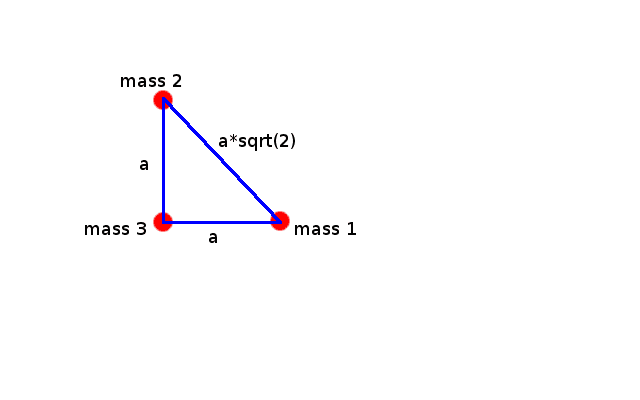# Finding the Normal Modes of a 3 mass molecule connected by springs

• VortexLattice
I don't remember what they are, but they're not real. Anyway, they're not real and don't enter into the solution.In summary, the goal of this homework is to find the normal modes of an equilibrium system, and the equations of motion. However, the problem becomes very nonlinear and difficult to solve.f

## Homework Statement

Hi everyone! I'm trying to solve a problem involving 3 equal masses (m) arranged in a 45-45-90 right triangle, each mass connected to the other two by a spring, and all the springs have the same spring constant (k). So it should look like this:Where I have made a the equilibrium length.

My goal is to set up the eigenvalue equation for its normal modes and find them.

## Homework Equations

The Euler Lagrange equations from the Lagrangian we will set up should lead to:

$\sum _{\lambda = 1} ^n (m_{σλ} \ddot{\eta}_\eta + v_{σλ}\eta _λ),\ \ σ = 1,2,...,n$

Where m is the mass matrix:

$m_{σλ} = \sum _i m_i (\frac{δx_i}{δq_σ})(\frac{δx_i}{δq_λ})$

Where the xi are our cartesian coordinates, and the q's are our generalized coordinates.

And v is the "potential matrix" (I couldn't find its real name):

$v_{σλ} = (\frac{δ^2 V}{δq_σ δq_λ})$

Where V is our potential.

We then guess that η is actually part of a complex number z, which would also be a solution if we had:

$η = Re(z)$

And we guess a solution for z:

$z = z_0 e^{iωt}$

And if we plug this into the first equation posted (for η, and differentiate, and divide by the exponent), we get an eigenvalue problem:

$\sum _{\lambda = 1} ^n (v_{σλ} - ω^2 m_{σλ}) z_λ ^0,\ \ σ = 1,2,...,n$

Here, zs is our eigenfunction corresponding to the eigenfrequency (value) ωs2.

## The Attempt at a Solution

First of all, I set up my generalized coordinates. We are looking at small oscillations, so I define for each mass:

$x_i = x_i ^0 + η_{ix}$

$y_i = y_i ^0 + η_{iy}$

Where x0 is the equilibrium distance for that coordinate and each η is the small deviation from the equilibrium length. The index of the masses are as shown in the picture.

So, first of all, we know that because we have 6 degrees of freedom, we will get 6 normal modes (though some of them (and are, because the problem said so) can be degenerate).

There seem to be two ways to do this problem, but I'm pretty sure they're actually the same way in the end. The first is to set up the Lagrangian, then use the E-L equations to get 6 equations, the equations of motion. These equations could then be expressed in matrix notation as

$(\underline{v} - \underline{m}ω^2)\underline{z_s}$

Where v and m are the previous matrices, and zs

On the other hand, we could just find the matrices m and v outright by their definitions above, rather than making the E-L equations match them.

Either way, from this matrix equation, we know solve for the determinant, and theoretically this should give us all the normal modes.

Using the above transformations, we quickly find the mass matrix to be diagonal, with m in each of the diagonal spots and zero otherwise:

$m_{ij} = mδ_{ij}$

Either way I do it, I have to find V (to differentiate the Lagrangian, or to just solve for the matrix v). But when I try to find V, I seem to get nonlinear stuff no matter what I try. This nonlinear stuff can be differentiated for sure, but when I do, it's messy and you can't get any answers from it.

To calculate the potential, we can add up the potentials from each spring separately. For the spring between mass 1 and mass 2, I'll call the potential V12, and so on.

So for spring 13, we call its equilibrium length a. Then, if it stretches and turns a little, its new length d is:

$d = \sqrt{(a + η_{1x} - η_{3x})^2 + (η_{1y} - η_{3y})^2}$

and

$V_{13} = \frac{k}{2}(d - a)^2$

And this is what I'm talking about...we don't even need to do the potentials for the other springs (though I did). The term with the square root just gets nastier as you differentiate it (twice) to find v. I assume I have to make some approximation to solve this, but I have no idea where... I tried assuming the springs don't turn while they're oscillating, and that makes the potentials V13 and V23 nice and simple (and solvable) but it still leaves V12 complicated and unsolvable.

Can anyone help me?? I'm at my wits end.

Thanks!

You can not spare those derivations, but at the end you should replace all η-s by zero in the second derivatives. Note that taking the derivative of V13=k/2(d-a)2 with respect to a variable u, it is k(d-a)du' and the second one with respect to v is kd'ud'v+k(d-a)d"uv. As your matrix elements are the second derivatives for zero displacements, the second term cancels (d-a=0). So you need to calculate the first derivatives of d only.

ehild

Erm...maybe that will work too, but I don't think that's really gets it all. I found that you have to use some approximations, notably

$(1 + x)^{1/2} \approx 1 + \frac{x}{2}$

And also say that powers of eta higher than 2nd order are negligible.